Courses

# NEET Previous Year Questions (2014-20): Electrostatics Potential and Capacitance Class 12 Notes | EduRev

## Class 12 : NEET Previous Year Questions (2014-20): Electrostatics Potential and Capacitance Class 12 Notes | EduRev

The document NEET Previous Year Questions (2014-20): Electrostatics Potential and Capacitance Class 12 Notes | EduRev is a part of the Class 12 Course Physics 28 Years Past year papers for NEET/AIPMT Class 12.
All you need of Class 12 at this link: Class 12

Q.1. In a certain region of space with volume 0.2 m3, the electric potential is found to be 5 V throughout. The magnitude of electric field in this region is:      (2020)
A: 1 N/C
B: 5 N/C
C: Zero
D: 0.5 N/C
Ans: C
given v = const. (5 volt)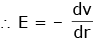E = 0

Q.2. The capacitance of a parallel plate capacitor with air as medium is 6 μF. With the introduction of a dielectric medium, the capacitance becomes 30 μF. The permittivity of the medium is :       (2020)
0 = 8.85×10-12 C2 N-1 m-2
A: 0.44×10-10 C2 N-1 m-2
B: 5.00 C2 N-1 m-2
C: 0.44×10-13 C2 N-1 m-2
D: 1.77×10-12 C2 N-1 m-2
Ans: A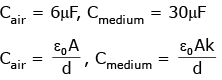Cmed = KCair
30μF = K6μF
K = 5
∴ ε = ε0k = 8.85 × 10-12 × 5
ε = 44.25 × 10-12
ε = 0.4425 × 10-10
ε = 0.44 × 10-10 C2N-1m-2

Q.3. A 40 μF capacitor is connected to a 200 V, 50 Hz ac supply. The rms value of the current in the circuit is, nearly:      (2020)
A: 2.5 A
B: 25.1 A
C: 1.7 A
D: 2.05 A
Ans:
A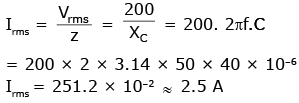Q.4. The electrostatic force between the metal plates of an isolated parallel plate capacitor C having a charge Q and area A, is :-     (2018)
A: independent of the distance between the plates.
B: linearly proportional to the distance between the plates
C: proportional to the square root of the distance between the plates.
D: inversely proportional to the distance between the plates.
Ans:
A
Solution:

F =  QE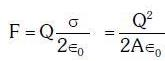∴ Electrostatic force is independent of distance between plates

Q.5. A capacitor is charged by a battery. The battery is removed and another identical uncharged capacitor is connected in parallel. The total electrostatic energy of resulting system :-   (2017)
A: Decreases by a factor of 2
B: Remains the same
C: Increases by a factor of 2
D: Increases by a factor of 4
Ans: A
Solution: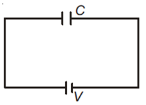Charge on capacitor
q = CV
when it is connected with another uncharged capacitor.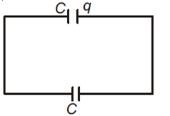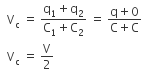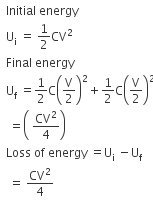Q.6. The diagrams below show regions of equipotentials:-     (2017)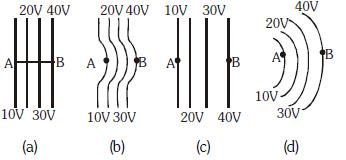A positive charge is moved from A to B in each diagram.
A: Maximum work is required to move q in figure (c)
B: Minimum work is required to move q in figure (a)
C: Maximum work is required to move q in figure (b)
D: In all the four cases the work done is the same
Ans:
D
Solution:
W = qΔV
as ΔV is same in all conditions, work will be same.

Q.7. A capacitor of 21F is charged as shown in the diagram. When the switch S is turned to position 2, the percentage of its stored energy dissipated is :    (2016)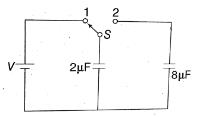A: 80%
B: 0%
C: 20%
D: 75%
Ans:
A
Solution:

Consider the figure given above.
When switch S is connected to point 1, then initial energy stored in the capacitor is given as,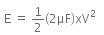When the switch S is connected to point 2, energy dissipated on connection across 8μF will be,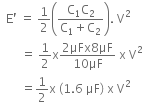Therefore, per centage loss of energy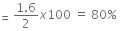Q.8. A parallel plate air capacitor of capacitance C is connected to a cell of emf V and then disconnected from it. A dielectric slab of dielectric constant K, which can just fill the air gap of the capacitor, is now inserted in it. Which of the following is incorrect ?     (2015)
A: The charge on the capacitor is not conserved.
B: The potential difference between the plates decreases K times
C: The energy stored in the capacitor decreases K times

D: the change in energy stored is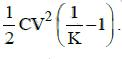Ans:
A
Solution:
A parallel plate air capacitor of capacitance C is connected to a cell of emf V and then disconnected from it.
The charge on the capacitor is given by
Q = CV
The energy stored in the capacitor is
E = 1/2 CV2
When a dielectric slab of dielectric constant K is inserted in it, the charge Q is conserved.
The capacitance becomes K times the original capacitance. (C' = KC)
The voltage becomes 1/K time the original voltage
V' = V/K
The change in energy stored is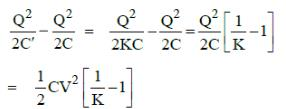Q.9. In a region, the potential is represented by V(x, y, z) = 6x − 8xy − 8y + 6yz, where V is in volts and x, y, z are in meters. The electric force experienced by a charge of 2 coulomb situated at point (1,1,1) is:     (2014)
A: 24N
B: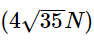C: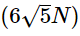D: 30N
Ans:
B
Solution: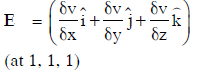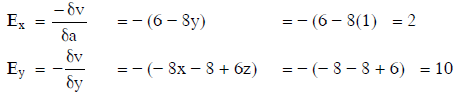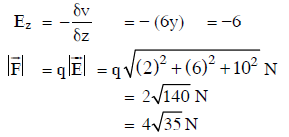Q.10. A conducting sphere of radius R is given a charge Q. The electric potential and the electric field at the centre of the sphere respectively are :    (2014)
A: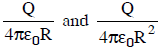B: Both are zero
C: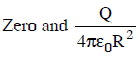D: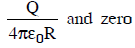Ans:
D
Solution: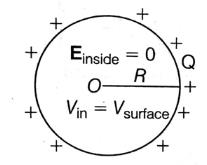Electric field inside, Einside = 0
Potential, Vinside = Vsurface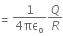Offer running on EduRev: Apply code STAYHOME200 to get INR 200 off on our premium plan EduRev Infinity!

14 docs|25 tests

,

,

,

,

,

,

,

,

,

,

,

,

,

,

,

,

,

,

,

,

,

;